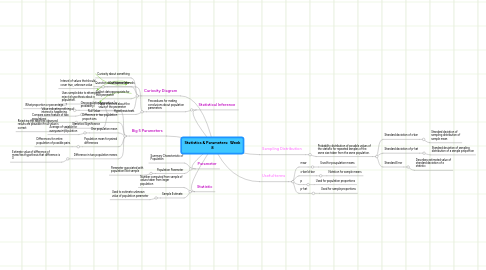Statistics & Parameters: Week 8

Get Started. It's FreeStatistics & Parameters: Week 81. Parameter

1.2. Population Parameter

1.2.1. Parameter associated with population/Not sample

2. Statistic

2.2. Sample Estimate

2.2.1. Used to estimate unknown value of population parameter

3. Statistical Inference

3.1. Preocedures for making conclusions about population parameters

3.1.1. Confidence Intervals

3.1.1.1. Interval of values that should cover true, unknown value

3.1.2. Hypothesis tests

3.1.2.1. Uses sample data to attempt to reject a hypothesis about a population.

3.1.2.2. Null Value

3.1.2.2.1. Value indicating nothing of interest is happening.

3.1.2.3. Statistical Significance

3.1.2.3.1. Rejecting the idea that observed results are plausible if null value is correct

5. Useful terms

5.1. mew

5.1.1. Used for poupulation means

5.2. x-bar/d-bar

5.2.1. Notation for sample means

5.3. p

5.3.1. Used for population proportions

5.4. p-hat

5.4.1. Used for sample proportions

6. Big 5 Parameters

6.1. One population proportion (or probability)

6.1.1. What proportion or percentage....?

6.2. Difference in two population proportions

6.2.1. Compare some feature of two populations

6.3. One population mean

6.3.1. Average of variable for everyone in population

6.4. Population mean for paired differences

6.4.1. Differences for entire population of possible pairs.

6.5. Difference in two population means

6.5.1. Estimate value of difference of mean/test hypothesis that difference is 0

7. Sampling Distribution

7.1. Probability distribution of possible values of the statistic for repeated samples of the same size taken from the same population.

7.1.1. Standard deviation of x-bar

7.1.1.1. Standard deviation of sampling distribution of sample mean

7.1.2. Standard deviation of p-hat

7.1.2.1. Standard deviation of sampling distribution of a sample proportion

7.1.3. Standard Error

7.1.3.1. Describes estimated value of standard deviation of a statistic